Get instant live expert help with Excel or Google Sheets“My Excelchat expert helped me in less than 20 minutes, saving me what would have been 5 hours of work!”

#### Post your problem and you’ll get expert help in seconds.

Your message must be at least 40 characters
Our professional experts are available now. Your privacy is guaranteed.

# How to Write an IF Statement in Excel

The IF function is one of the most popular and widely used functions in Excel. This function is used to test logical comparisons or conditions on a value. This is a powerful feature once you’ve learned the basics of constructing a statement.

## How to Write an IF statement in Excel

The IF statement has two parts; first IF a comparison or condition is TRUE, and second IF a comparison or condition is FALSE.

In Excel it has following syntax to follow;

`=IF (logical_test, [value_if_true], [value_if_false])`

Where,

logical_test – It is logical comparison or condition that is tested as TRUE or FALSE.

value_if_true – It is a value to be returned when logical_test is TRUE. It is optional.

value_if_false – It is a value to be returned when logical_test is FALSE. It is also optional.

By following this syntax, you perform a logical test or condition on a value, and return a specified value if logical test is TRUE, else return another value if logical test is FALSE.

In simple words, it says, that if a number is greater than a certain number, then return “Pass,” otherwise return “Fail.

### Some Rules to follow with the IF function

While using the IF function, you need to follow a few rules.

• If you forget to supply the value_if_true argument in the IF function and use only a comma followed by the logical_test argument, then the IF function returns zero (0) for the True argument part, such as;`=IF(B2>60,,"Bad")`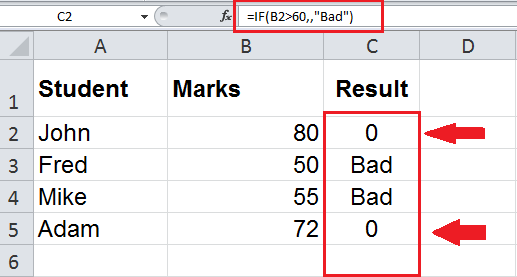• If you don’t supply the value_if_false argument, and use only a closing bracket followed by the value_if_true argument, then the IF function returns FALSE, such as;`=IF(B2>60,"Good")`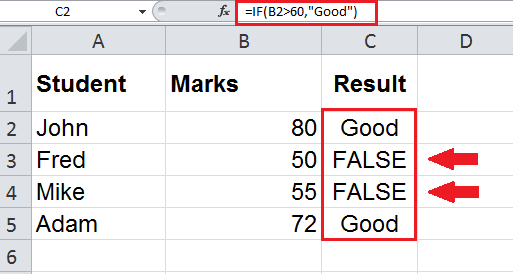• If you want to return numeric values or logical test values as TRUE and FALSE in value_if_true and value_it_false arguments, then you do not need to enclose in double quotation marks, such as;`=IF(B2>60,1,0)`

OR

`=IF(B2>60,TRUE,FALSE)`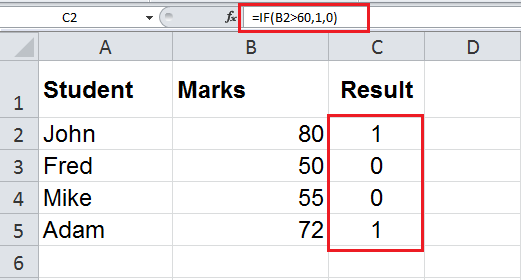OR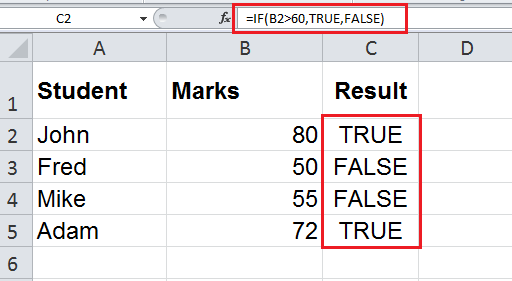• Based on a logical_test result you can perform calculations as per your requirement in value_if_true and value_if_false arguments of IF functions, such as;`=IF(B2>3000,B2*2%,B2*1%)`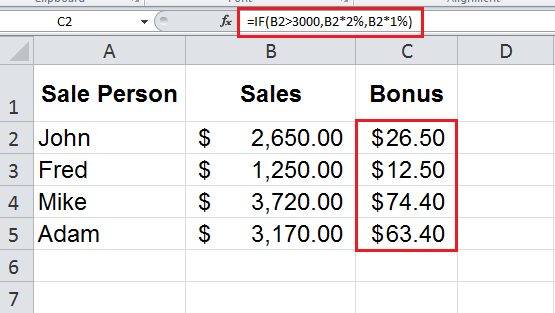### The IF function for Comparing Numbers

Numbers are always logically tested using comparison operators in IF function, like Greater Than (>), Greater Than Equal to (>=), Less Than (<), Less Than Equal to (<=), Equal To (=), Not Equal to (<>) and based on TRUE and FALSE results some specified values are returned.

For example, you have sales data of salespersons for a particular month and you need to compare each salesperson’s sales amount against average monthly sales and return resulting values, like “Above Average” or “Below Average”.

You need to calculate the average monthly sales using the AVERAGE function for comparison purpose, say in cell B2, and you need to compare each salesperson’s sales amount with this average monthly sales figure using IF function, such as;

`=IF(B5>\$B\$2,"Above Average","Below Average")`

You need to make the cell reference of average monthly sales as an absolute value by using \$ sign in the above IF statement so that this cell reference does not change when you copy the formula to other rows.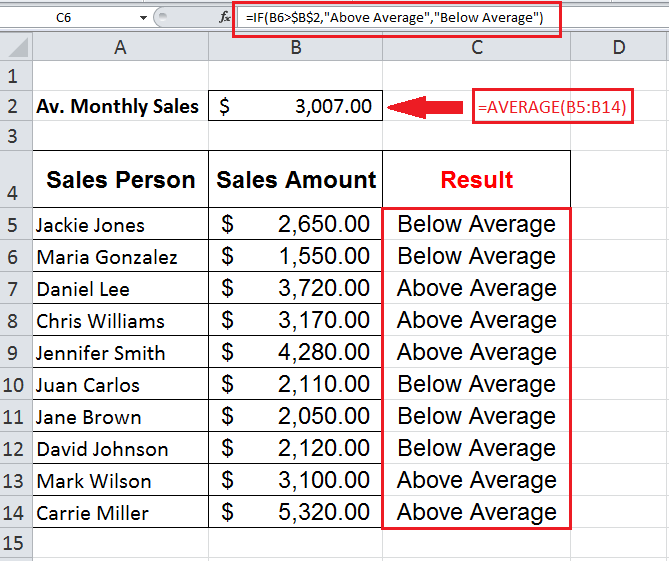You can also introduce other functions in the IF statement to make calculations like the AVERAGE function. So instead of calculating the average monthly sales amount in a separate cell for comparison, you can also calculate the average using the AVERAGE function within an IF statement like;

`=IF(B5>AVERAGE(\$B\$5:\$B\$14),"Above Average","Below Average")`

You need to make the cells range B5:B14 an absolute range by using \$ sign with column and row references, so that it does not change when you copy the formula to other rows as shown above.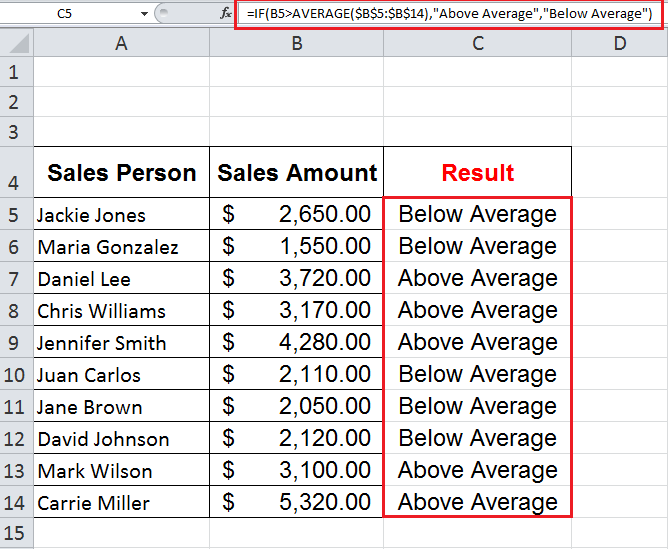### The IF function for Text values

Text values in the IF function are logically tested using the comparison operators Equal to (=) or Not Equal to (<>) and based on TRUE and FALSE results, specified resulting values are returned.

For example, you need to compare the status of each order, and based on TRUE and FALSE condition arguments you need to return “Done” and “Check Status” values using IF statement, such as;

`=IF(G4="Delivered","Done","Check Status")`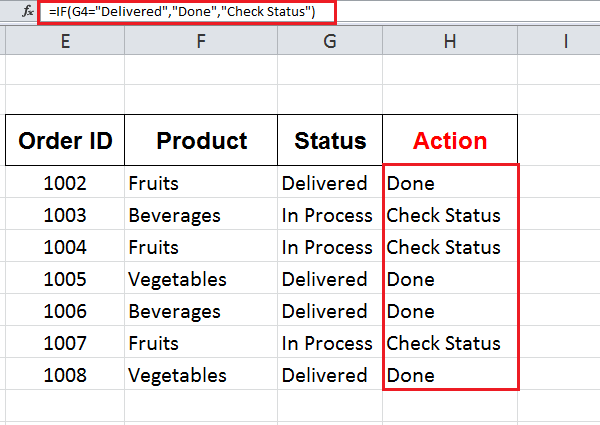### The IF function for Date values

Like numbers, date values are also logical tested using comparison operators in the IF function, like Greater Than (>), Greater Than Equal to (>=), Less Than (<), Less Than Equal to (<=), Equal To (=), Not Equal to (<>) and specified values are returned where logical test returns TRUE and FALSE.

For example, you have data for various orders to various companies and you have an expected delivery date and actual delivery date for each order. You want to test if the difference between the actual delivery date and expected delivery date is greater than or equal to 7 days then mark that order “Late”, otherwise mark it as “In-Time”, such as;

`=IF(M3-L3>=7,"Late", "In-Time")`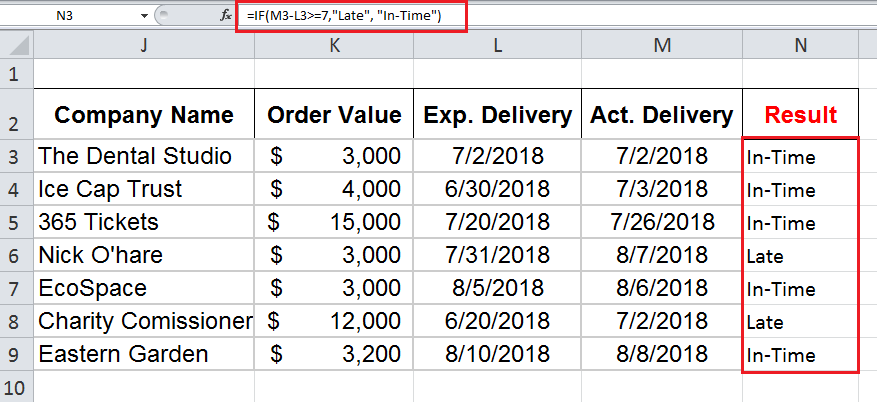You can also use other date functions like TODAYDATE or DATEVALUE within a logical_test argument of the IF statement to test the condition and return the values as per your requirement.

For example, you want to test if the expected delivery date is approaching nearer then you want to return an alert value “Check progress”, otherwise you want to keep an eye on the delivery status of an order. If the difference between an expected delivery date and Today’s date is greater than equal to 1, then return “Check Progress”, else return “Delivered or Not?

`=IF(L3-TODAY()>=1,"Check Progress","Delivered or Not?")`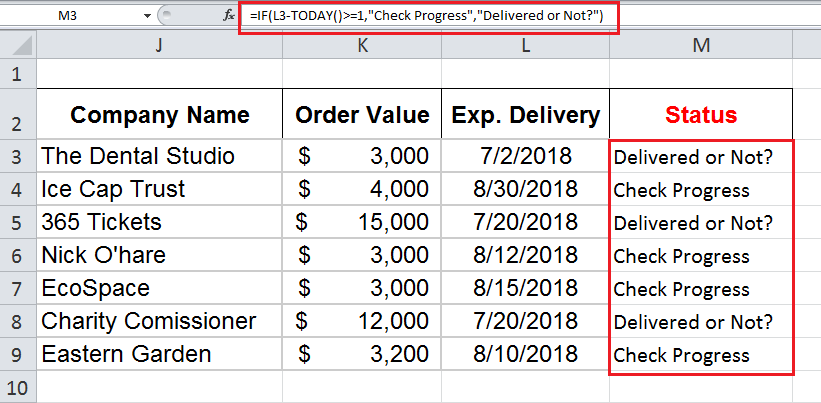Still need some help with Excel formatting or have other questions about Excel? Connect with a live Excel expert here for some 1 on 1 help. Your first session is always free.

Are you still looking for help with the IF function? View our comprehensive round-up of IF function tutorials here.

### Did this post not answer your question? Get a solution from connecting with the expert.Another blog reader asked this question today on Excelchat:
Related blogs
Solution examplescan you teach me the steps to create a nested if function, and to nest an AND function inside of an IF function?
Solved by A. Q. in 22 minsI have a list or people who are ranked in numbers from 3 to 6 I need to recognize the contents of each multiple cells and create an equivalent letter. for that value into another column. e.g. Cell E1 = 3 to show in new cell that row (H1) the letter "C" I have multiple rows with different values in column "E" Can this be done?
Solved by I. J. in 30 minsI just need to edit this formula, {=IFERROR(AVERAGEIFS(B6:D6,F6:H6,"FALSE"),AVERAGEIF(B6:D6,"<>0"))}, to return "-" or "0" if there is nothing in column D. I can share the entire worksheet if need be.
Solved by V. A. in 47 minsCould you help me by explaining the IF function?
Solved by G. L. in 24 minsI want to display a range of cells if they meet a specific criteria. I want to display a name IF it does not equal one of three options AND there are any numbers contained in a range of cells
Solved by G. F. in 60 mins## Subscribe to Excelchat.coAnother blog reader asked this question today on Excelchat: